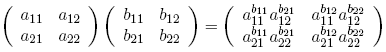A nonassociative array product is naturally defined by replacing multiplication with power and addition with multiplication, as in the $2 \times 2$ caseObserve that matrices which are magic under normal matrix multiplication have analogues which are magic under these power products, in the sense that the multiplication of entries along each row and column is equal to some constant. For example, the analogue of the usual democratic matrixis the matrix with entries the cubed root of unity. Note that the identity matrix still acts as an identity, but we will not bother to define a zero element, because we don't much care if things turn out to be like ordinary fields or not.
Demanding a magic sum of 1, and a magic product of 1 in the new nonassociative algebra, results in a mapping of the positive real interval $[0,1]$ to the complex unit circle. Scalar multiples do not exist in the new array product. If we replace zero by the number 1, then all permutation matrices must be mapped to the (power) democratic matrix with unit entries, namely three times the original democratic matrix.